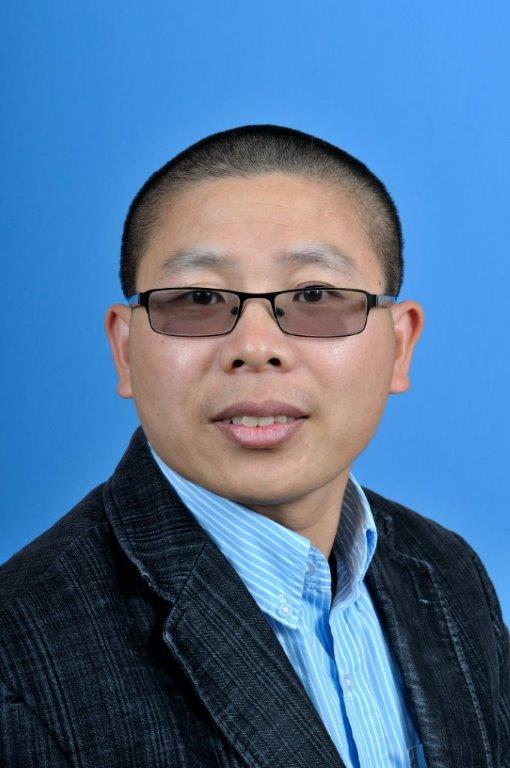# Dr. Xin TangProfessor in Mathematics

Office:  Science & Technology 424

Phone:  (910) 672-2206

Email: xtang@uncfsu.edu

Personal Homepage:

BIOGRAPHY

Graduated from Kansas State University in May 2006 with a Ph.D. degree in Mathematics. Joined Fayetteville State University in August 2006.

TEACHING

Math 121-Introduction to College Algebra
Math 123-College Algebra
Math 126-Quantative Reasoning
Math 129-PreCalculus I
Math 130-PreCalculus II
Math 131-Algebra and Trigonometry
Math 140-Applied Calculus
Math 142-Calculus with Analytical Geometry I
Math 150-Discrete Mathematics I
Math 241-Calculus with Analytic Geometry II
Math 242-Calculus with Analytic Geometry III
Math 251-Linear Algebra
Math 361-Introduction to Modern Algebra I
Math 362-Introduction to Modern Algebra II
Math 472-Theory of Numbers
Math 481-Introduction to Topology

RESEARCH INTERESTS

Noncommutative Algebraic Geometry and Representation Theory
Derivations and Endomorphisms for Quantum Algebras
Hopf Actions and Cancellation Problems for Quantum Algebras

SELECTED PUBLICATIONS

Tang, XinZhang, James J.Zhao, Xiangui Cancellation of Morita and skew types. Israel J. Math. 244 (2021), no. 1, 467-500.
Tang, XinVenegas Ramírez, Helbert J.Zhang, James J. Cancellation problem for AS-regular algebras of dimension three. Pacific J. Math. 312 (2021), no. 1, 233-255.
Tang, Xin The automorphism groups for a family of generalized Weyl algebras. J. Algebra Appl. 17 (2018), no. 8, 1850142, 20 pp.
Tang, Xin Automorphisms for some symmetric multiparameter quantized Weyl algebras and their localizations. Algebra Colloq. 24 (2017), no. 3, 419-438.
Tang, Xin Down-up algebras over a polynomial base ring K[t1,...,tn]. J. Algebra Appl. 15 (2016), no. 2,1650024, 16 pp.
Tang, Xin Algebra endomorphisms and derivations of some localized down-up algebras. J. Algebra Appl.14 (2015), no. 3, 1550034, 14 pp.
Tang, Xin Derivations of the two-parameter quantized enveloping algebra U+r,s(B2). Comm. Algebra 41 (2013), no. 12, 4602-4621
Tang, Xin (Hopf) algebra automorphisms of the Hopf algebra Uˇ≥0r,s(sl3). Comm. Algebra 41 (2013), no. 8, 2996-3012.
Tang, Xin Mad subalgebras and Lie subalgebras of an enveloping algebra. Bull. Aust. Math. Soc. 82 (2010), no. 3, 401-423
Tang, Xin Ringel-Hall algebras and two-parameter quantized enveloping algebras. Pacific J. Math. 247 (2010), no. 1, 213-240.
Xu, Yun GeTang, Xin Hochschild (co)homology of Galois coverings of Grassmann algebras. Acta Math. Sin. (Engl. Ser.) 25 (2009), no. 10, 1693-1702.
Tang, XinXu, Yunge On representations of quantum groups Uq(fm(K,H)). Bull. Aust. Math. Soc. 78 (2008), no. 2, 261-284.
Tang, Xin Construct irreducible representations of quantum groups Uq(fm(K)). Front. Math. China (2008), no. 3, 371-397.
Tang, Xin On irreducible weight representations of a new deformation Uq(sl2) of U(sl2). J. Gen. Lie Theory Appl. (2007), no. 2, 97-105.
Tang, Xin On Whittaker modules over a class of algebras similar to U(sl2). Front. Math. China (2007), no. 1, 127-142.
Tang, XinWu, Qiuxian Nakayama algebras and syzygy. Beijing Shifan Daxue Xuebao 35 (1999), no. 4,443-446.